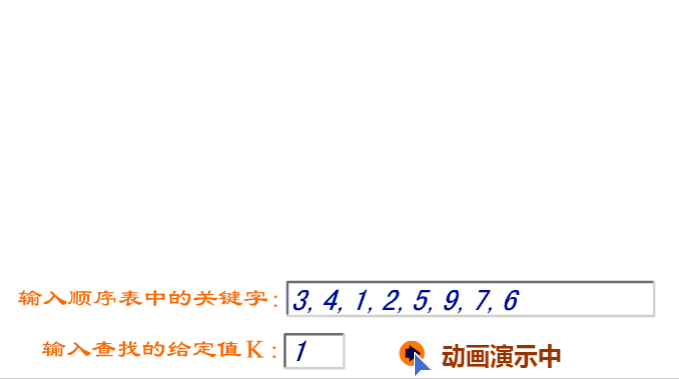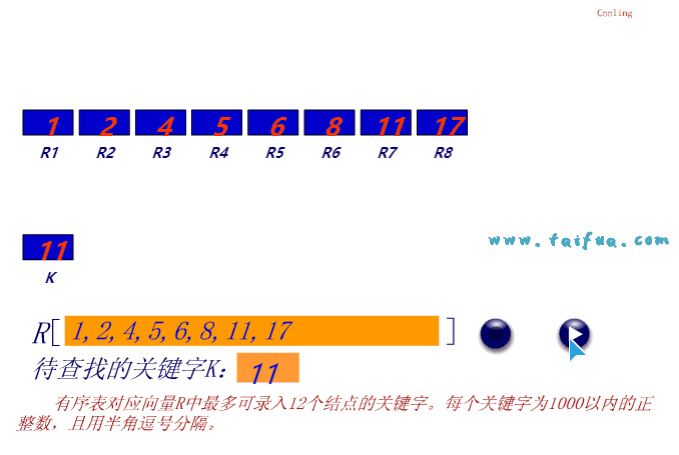# 数据结构笔记（七）：线性表的查找之顺序查找与二分查找

## 顺序查找

### 效果演示

`3,4,1,2,5,9,7,6`中寻找`1`的位置。### 算法实现

``````#include <iostream>
using namespace std;

int search1(int a[], int n, int key) // 顺序查找
{
a = key; // 监视哨
int i;
for (i = n; a[i] != key; --i) // 从第n个元素开始逆序查找
;
return i; // 返回0表示查询失败
}

int search2(int a[], int n, int key) // 同上
{
a = key; // 监视哨
int i = n;
while(a[i] != key)
--i;
return i;
}

int main()
{
int n;
int a;
cin >> n;
for (int i = 1; i <= n; ++i)
cin >> a[i];
int key; // 查询的值
while(cin >> key)
{
cout << search1(a, n, key) << endl; // 输出查询位置
cout << search2(a, n, key) << endl;
}
return 0;
}
``````
``````8 // 输入长度为8的数组
3 4 1 2 5 9 7 6
4 // 查找4返回2（查询成功）
2
2
5 // 查找5返回5（查询成功）
5
5
9 // 查找9返回6（查询成功）
6
6
10 // 查找10返回0（查询失败）
0
0
``````

## 二分查找

### 基本知识

• 存储在数组中
• 有序排列

• `a[left..right]`是当前的查找区间
• 首先确定该区间的中点位置：`mid = (left+right) / 2`，即`mid = (left+right) >> 1`，有时候也写成`left + ((right-left) >> 1)`，不解释。
• 然后将待查的`Key`值与`a[mid]`比较：若相等，则查找成功并返回此位置，否则须确定新的查找区间，继续二分查找。
• ①若`a[mid]>Key`，则由表的有序性可知a[mid..n]均大于Key，因此若表中存在关键字等于Key的结点，则该结点必定是在位置mid左边的子表a[1..mid-1]中，故新的查找区间是左子表a[1..mid-1]。
• ②若`a[mid]<Key`，则要查找的Key必在mid的右子表a[mid+1..n]中，即新的查找区间是右子表a[mid+1..n]。
• 因此，从初始的查找区间a[1..n]开始，每经过一次与当前查找区间的中点位置上的结点关键字的比较，就可确定查找是否成功，不成功则当前的查找区间就缩小一半。这一过程重复直至找到关键字为K的结点，或者直至当前的查找区间为空(即查找失败)时为止。

### 效果演示

`1, 2, 4, 5, 6, 8, 11, 17`寻找`11`的位置。### 算法实现

• 查询数组元素位置从0开始返回-1表示未找到
``````#include <iostream>
#include <algorithm>
using namespace std;

int Binary_Search(int a[], int n, int key) // 数组下标从0开始
{
int left = 0, right = n - 1;
while (left <= right)
{
int mid = left + ((right-left) >> 1);
if (a[mid] < key)
left = mid + 1;
else if (a[mid] > key)
right = mid - 1;
else
return mid;
}
return -1; // 返回-1表示未找到
}

int main()
{
int a = {1, 2, 4, 5, 6, 8, 11, 17}; // 下标从0开始
int n = 8; // 数组元素数量
int m = 8; // 查询次数
int key; // 查询的元素
while (m--)
{
cin >> key;
cout << Binary_Search(a, n, key)+1 << endl; // 输出实际位置+1
}
return 0;
}
/*
1 // 查询1
1
2 // 查询2
2
4 // 查询4
3
5 // 查询5
4
6 // 查询6
5
8
6
11
7
17
8
*/
``````
• 查询数组元素位置从1开始返回0表示未找到
``````int Binary_search(int a[], int n, int x) // 数组下标从1开始
{
int left = 1, right = n;
while (left <= right)
{
int mid = (left+right) >> 1; // 二分
if (a[mid] < x)
left = mid + 1;
else if (a[mid] > x)
right = mid - 1;
else
return mid; // 查询到返回元素索引(下标)
}
return 0; // 返回0表示未找到
}
``````
• 二分搜索递归版本
``````int _Binary_Search(int a[], int left, int right, int key)
{
if (left > right)
return -1;
int mid = (left + right) >> 1;
if (a[mid] > key)
return _Binary_Search(a, left, mid - 1, key);
if (a[mid] < key)
return _Binary_Search(a, mid + 1, right, key);
return mid;
}

_Binary_Search(a, 1, n, key) // 调用
``````

### 算法分析

• 查找有序数组中第一个与key相等的元素
• 查找有序数组中最后一个与key相等的元素
• 查找数组中某个数出现的次数

• 使用二分查询有序数组a中大于等于x的第一个数的位置（数组下标从1开始）
``````int Binary_search1(int a[], int n, int x)
{
int l = 1, r = n;
while (l <= r)
{
int m = (l+r) >> 1;
if (a[m] < x)
l = m + 1;
else
r = m - 1;
}
return r + 1;
}
``````
• 使用二分查询有序数组a中小于等于x的最后一个数的位置（数组下标从1开始）
``````int Binary_search2(int a[], int n, int x)
{
int l = 1, r = n;
while (l <= r)
{
int m = (l+r) >> 1;
if (a[m] <= x)
l = m + 1;
else
r = m - 1;
}
return l - 1;
}
``````

### 补充

C++ STL 自带`binary_search()`二分查找以及`sort()`排序。但是请注意，binary_search() 用来在一个有序区间中使用二分法查找元素是否在这个区间中，它的返回值为bool，不是下标位置

``````int a = {1, 7, 3, 5, 9, 6, 2, 4};
sort(a, a + 8); //首先排序
bool f = binary_search(a， a+n, key); // 然后使用二分查找
// 返回值为false表示未找到，为true表示找到
``````

``````void Swap(int & a, int & b) // 交换两数
{
int t = a;
a = b;
b = t;
}

void bubble_sort(int a[], int n) // 冒泡排序
{
for (int i = 0; i < n-1; ++i)
for (int j = i + 1; j < n; ++j)
if (a[i] > a[j]) // 从小到大排序
Swap(a[i], a[j]);
}
int a = {1, 7, 3, 5, 9, 6, 2, 4};

bubble_sort(a, n);
``````

The end.
2017-11-24 星期五

### 更新

• 代码
``````// 下标从1开始，找到第一个大于等于x的值的位置
int Binary_search1(int a[], int n, int x)
{
int l = 1, r = n;
while (l <= r)
{
int m = (l+r) >> 1;
if (a[m] < x)
l = m + 1;
else
r = m - 1;
}
return r + 1;
}

// 下标从1开始，找到最后一个小于等于x的值的位置
int Binary_search2(int a[], int n, int x)
{
int l = 1, r = n;
while (l <= r)
{
int m = (l+r) >> 1;
if (a[m] <= x)
l = m + 1;
else
r = m - 1;
}
return l - 1;
}

// 下标从0开始，找到第一个大于x的值的位置
int binary_Search1(int a[], int n, int val)
{
int l = 0, r = n - 1;
while (l <= r)
{
int m = (l+r) >> 1;
if (a[m] <= val)
l = m + 1;
else
r = m - 1;
}
return r + 1;
}

// 下标从0开始，找到最后一个小于x的值的位置
int binary_Search2(int a[], int n, int val)
{
int l = 0, r = n - 1;
while (l <= r)
{
int m = (l+r) >> 1;
if (a[m] < val)
l = m + 1;
else
r = m - 1;
}
return l - 1;
}
``````
• 调用
``````int a = {1, 2, 2, 2, 3, 5, 6, 7, 7, 7, 9, 9};
int b = {0, 1, 2, 2, 2, 3, 5, 6, 7, 7, 7, 9, 9};
int x;
while (cin >> x)
{
cout << "下标从0开始：" << endl;
cout << binary_Search1(a, 12, x) << " " << binary_Search2(a, 12, x) << endl;
cout << "下标从1开始：" << endl;
cout << Binary_search2(b, 12, x) << " " << Binary_search1(b, 12, x) << endl;
}
``````
• 结果
``````1
1 -1
1 1
2
4 0
4 2
7
10 6
10 8
9
12 9
12 11
``````

The end.
2017-12-05 星期二

1. 太傅 fucking great

1. @ZJing

谢谢捧场哇！

2. 欧巴桑好厉害哦，代码颜色换一下啊，狗眼瞎了

1. @CongTsang换了换了